# `sklearn.gaussian_process.kernels`.RBF¶

class `sklearn.gaussian_process.kernels.``RBF`(length_scale=1.0, length_scale_bounds=(1e-05, 100000.0))[source]

Radial-basis function kernel (aka squared-exponential kernel).

The RBF kernel is a stationary kernel. It is also known as the “squared exponential” kernel. It is parameterized by a length-scale parameter length_scale>0, which can either be a scalar (isotropic variant of the kernel) or a vector with the same number of dimensions as the inputs X (anisotropic variant of the kernel). The kernel is given by:

k(x_i, x_j) = exp(-1 / 2 d(x_i / length_scale, x_j / length_scale)^2)

This kernel is infinitely differentiable, which implies that GPs with this kernel as covariance function have mean square derivatives of all orders, and are thus very smooth.

New in version 0.18.

Parameters: length_scale : float or array with shape (n_features,), default: 1.0 The length scale of the kernel. If a float, an isotropic kernel is used. If an array, an anisotropic kernel is used where each dimension of l defines the length-scale of the respective feature dimension. length_scale_bounds : pair of floats >= 0, default: (1e-5, 1e5) The lower and upper bound on length_scale

Methods

 `clone_with_theta`(theta) Returns a clone of self with given hyperparameters theta. `diag`(X) Returns the diagonal of the kernel k(X, X). `get_params`([deep]) Get parameters of this kernel. `is_stationary`() Returns whether the kernel is stationary. `set_params`(\*\*params) Set the parameters of this kernel.
`__init__`(length_scale=1.0, length_scale_bounds=(1e-05, 100000.0))[source]
`bounds`

Returns the log-transformed bounds on the theta.

Returns: bounds : array, shape (n_dims, 2) The log-transformed bounds on the kernel’s hyperparameters theta
`clone_with_theta`(theta)[source]

Returns a clone of self with given hyperparameters theta.

`diag`(X)[source]

Returns the diagonal of the kernel k(X, X).

The result of this method is identical to np.diag(self(X)); however, it can be evaluated more efficiently since only the diagonal is evaluated.

Parameters: X : array, shape (n_samples_X, n_features) Left argument of the returned kernel k(X, Y) K_diag : array, shape (n_samples_X,) Diagonal of kernel k(X, X)
`get_params`(deep=True)[source]

Get parameters of this kernel.

Parameters: deep: boolean, optional : If True, will return the parameters for this estimator and contained subobjects that are estimators. params : mapping of string to any Parameter names mapped to their values.
`hyperparameters`

Returns a list of all hyperparameter specifications.

`is_stationary`()[source]

Returns whether the kernel is stationary.

`n_dims`

Returns the number of non-fixed hyperparameters of the kernel.

`set_params`(**params)[source]

Set the parameters of this kernel.

The method works on simple kernels as well as on nested kernels. The latter have parameters of the form `<component>__<parameter>` so that it’s possible to update each component of a nested object.

Returns: self :
`theta`

Returns the (flattened, log-transformed) non-fixed hyperparameters.

Note that theta are typically the log-transformed values of the kernel’s hyperparameters as this representation of the search space is more amenable for hyperparameter search, as hyperparameters like length-scales naturally live on a log-scale.

Returns: theta : array, shape (n_dims,) The non-fixed, log-transformed hyperparameters of the kernel

## Examples using `sklearn.gaussian_process.kernels.RBF`¶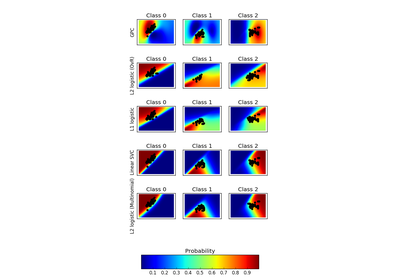Plot classification probability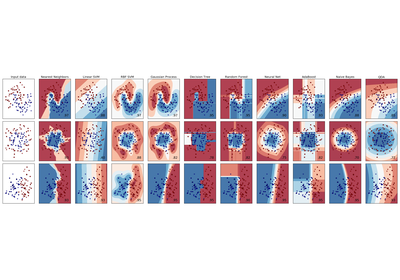Classifier comparison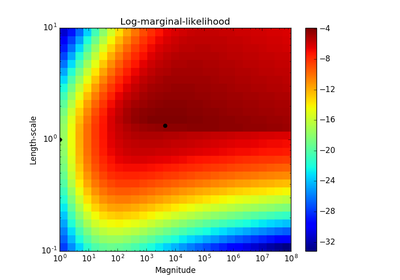Probabilistic predictions with Gaussian process classification (GPC)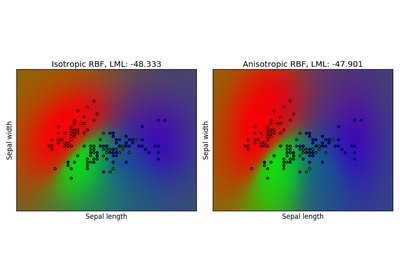Gaussian process classification (GPC) on iris dataset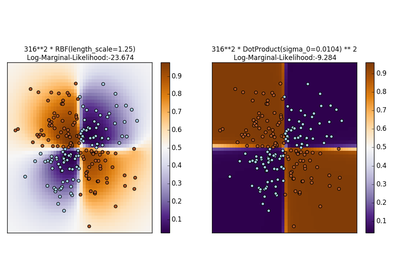Illustration of Gaussian process classification (GPC) on the XOR dataset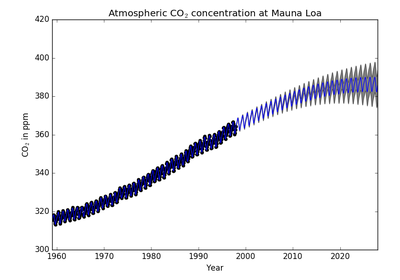Gaussian process regression (GPR) on Mauna Loa CO2 data.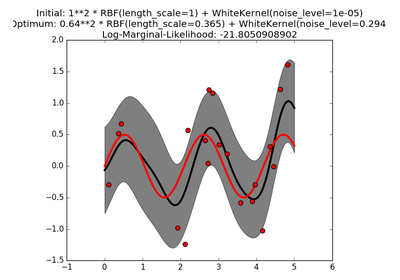Gaussian process regression (GPR) with noise-level estimation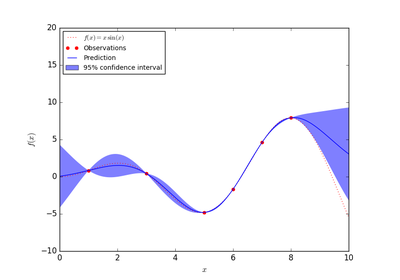Gaussian Processes regression: basic introductory example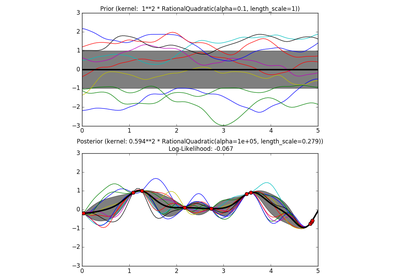Illustration of prior and posterior Gaussian process for different kernels